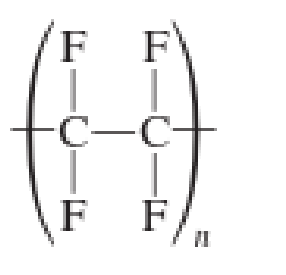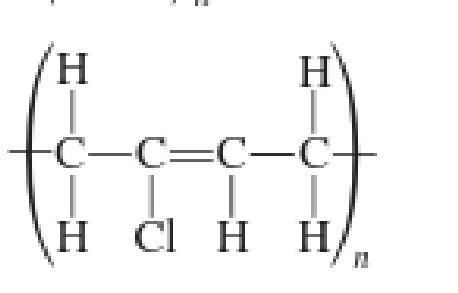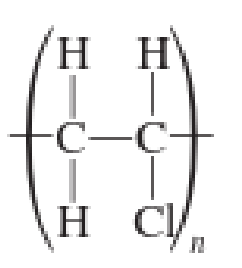Chapter 2, Problem 2.83EP### Organic And Biological Chemistry

7th Edition
STOKER + 1 other
ISBN: 9781305081079

#### Solutions

Chapter
Section### Organic And Biological Chemistry

7th Edition
STOKER + 1 other
ISBN: 9781305081079
Textbook Problem

# Draw the structural formula of the monomer(s) from which each of the following polymers was made.(a)

Interpretation Introduction

Interpretation:

The structural formula for the monomer unit from which the given polymer is formed has to be drawn.

Concept Introduction:

Polymer is a very large molecule that is formed by repetitive bonding together of many smaller molecules. The small repeating units are known as monomer. Polymerization is the process by which polymer is prepared.

Addition polymer is the one in which the monomers simply add together with no other products formed apart from the polymer. The repeating unit in addition polymer often exceeds 100,000 and sometimes can exceed even a million.

Explanation

Addition polymers are formed by repeated addition of monomer unit. The number of monomer units that are present in addition polymer often exceeds more than 100,000 and sometimes even a million.

(b)

Interpretation Introduction

Interpretation:

The structural formula for the monomer unit from which the given polymer is formed has to be drawn.

Concept Introduction:

Polymer is a very large molecule that is formed by repetitive bonding together of many smaller molecules. The small repeating units are known as monomer. Polymerization is the process by which polymer is prepared.

Addition polymer is the one in which the monomers simply add together with no other products formed apart from the polymer. The repeating unit in addition polymer often exceeds 100,000 and sometimes can exceed even a million.

(c)

Interpretation Introduction

Interpretation:

The structural formula for the monomer unit from which the given polymer is formed has to be drawn.

Concept Introduction:

Polymer is a very large molecule that is formed by repetitive bonding together of many smaller molecules. The small repeating units are known as monomer. Polymerization is the process by which polymer is prepared.

Addition polymer is the one in which the monomers simply add together with no other products formed apart from the polymer. The repeating unit in addition polymer often exceeds 100,000 and sometimes can exceed even a million.

(d)

Interpretation Introduction

Interpretation:

The structural formula for the monomer unit from which the given polymer is formed has to be drawn.

Concept Introduction:

Polymer is a very large molecule that is formed by repetitive bonding together of many smaller molecules. The small repeating units are known as monomer. Polymerization is the process by which polymer is prepared.

Addition polymer is the one in which the monomers simply add together with no other products formed apart from the polymer. The repeating unit in addition polymer often exceeds 100,000 and sometimes can exceed even a million.

### Still sussing out bartleby?

Check out a sample textbook solution.

See a sample solution

#### The Solution to Your Study Problems

Bartleby provides explanations to thousands of textbook problems written by our experts, many with advanced degrees!

Get Started